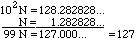Name: Kavoos Bybordi Who is asking: Student Level: Secondary Question: I dont know how to change a reccuring decimal to a fraction please could you tell me the method. Hi Kavoos, Suppose that we wished to write the repeating decimal N = 1.282828 ... in the form p/q where p and q are integers. Since the period of the repeating part is 2 (the 28 is being repeated) we look at 102N. Subtracting N from 102N givesand therefore 99 N = 127 and thus N = 1.282828 ... = 127/99 Cheers, Penny

Go to Math Central